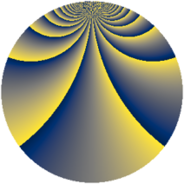# Properties

 Label 1520.2.ecLevel $1520$ Weight $2$ Character orbit 1520.ec Rep. character $\chi_{1520}(187,\cdot)$ Character field $\Q(\zeta_{36})$ Dimension $2832$ Sturm bound $480$

# Related objects

## Defining parameters

 Level: $$N$$ $$=$$ $$1520 = 2^{4} \cdot 5 \cdot 19$$ Weight: $$k$$ $$=$$ $$2$$ Character orbit: $$[\chi]$$ $$=$$ 1520.ec (of order $$36$$ and degree $$12$$) Character conductor: $$\operatorname{cond}(\chi)$$ $$=$$ $$1520$$ Character field: $$\Q(\zeta_{36})$$ Sturm bound: $$480$$

## Dimensions

The following table gives the dimensions of various subspaces of $$M_{2}(1520, [\chi])$$.

Total New Old
Modular forms 2928 2928 0
Cusp forms 2832 2832 0
Eisenstein series 96 96 0

## Trace form

 $$2832q - 12q^{2} - 24q^{3} - 12q^{4} - 12q^{5} - 24q^{6} - 12q^{7} - 6q^{8} + O(q^{10})$$ $$2832q - 12q^{2} - 24q^{3} - 12q^{4} - 12q^{5} - 24q^{6} - 12q^{7} - 6q^{8} - 12q^{10} - 12q^{11} - 6q^{12} - 12q^{16} - 24q^{17} - 24q^{18} - 24q^{20} - 24q^{21} - 24q^{23} + 36q^{24} + 36q^{26} - 12q^{27} - 6q^{30} - 42q^{32} - 24q^{33} - 48q^{34} - 42q^{35} - 72q^{36} + 90q^{38} + 72q^{40} + 24q^{42} - 6q^{45} - 12q^{46} - 72q^{47} - 96q^{48} - 6q^{50} - 48q^{51} - 12q^{52} - 24q^{53} - 48q^{54} - 24q^{55} - 48q^{56} - 168q^{58} - 78q^{60} - 24q^{61} + 36q^{62} + 48q^{64} - 12q^{65} - 24q^{66} - 6q^{68} - 48q^{69} - 12q^{70} - 48q^{71} - 144q^{72} - 48q^{74} - 192q^{75} - 24q^{76} + 120q^{77} + 102q^{78} + 6q^{80} - 48q^{81} + 228q^{82} - 12q^{83} + 72q^{84} - 12q^{85} - 24q^{86} - 12q^{87} - 102q^{88} - 42q^{90} - 24q^{91} - 12q^{92} - 48q^{94} - 120q^{95} - 144q^{96} - 24q^{97} - 66q^{98} + O(q^{100})$$

## Decomposition of $$S_{2}^{\mathrm{new}}(1520, [\chi])$$ into newform subspaces

The newforms in this space have not yet been added to the LMFDB.